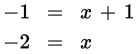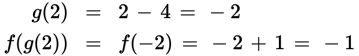# SAT Math Multiple Choice Question 345: Answer and Explanation

### Test Information

Question: 345

15. If f(g(2)) = -1 and f(x) = x + 1, then which of the following could define g(x)?

• A. g(x) = x – 6
• B. g(x) = x – 4
• C. g(x) = x – 2
• D. g(x) = x – 1

Explanation:

B

Difficulty: Hard

Category: Passport to Advanced Math / Functions

Strategic Advice: Understanding the language of functions will make questions that seem complicated much more doable on Test Day. When you know the output of a function (or in this question, a composition of two functions), you can work backward to find the input.

Getting to the Answer: Because g(x) is the inside function for this composition, its output becomes the input for f(x). Unfortunately, you don't have any information about g yet. You do know however that f of some number, (g(2)), is -1, so set f(x) equal to -1 and solve for x:You now know that f(-2) = -1. In the equation for the composition, g(2) represents x, so you also know that g(2) must be -2. Your only option now is to use brute force to determine which equation for g, when evaluated at 2, results in -2.

Choice A: g(2) = 2 - 6 = -4 (not -2), so eliminate.

Choice B: g(2) = 2 - 4 = -2

You don't need to go any further; (B) is correct.